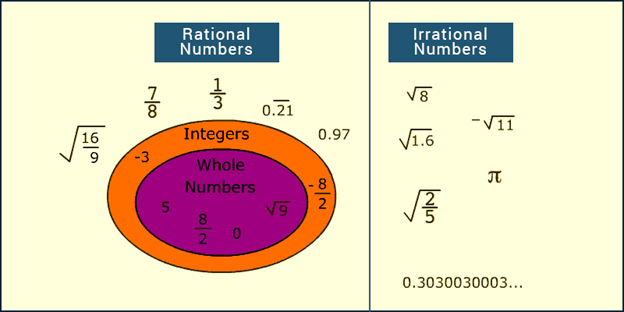# Irrational Numbers

Irrational numbers are the numbers that can be represented as fractions. It is a contradiction of rational numbers but are a type of real numbers. Hence, we can represent it as R\Q, where the backward slash symbol denotes ‘set minus’ or it can also be denoted as R – Q, which means set of real numbers minus set of rational numbers. The calculations based on these numbers are a bit complicated. For example, √5, √11, √21, etc are irrational. If such numbers are used in arithmetic operations, then first we need to evaluate the values under root. These values could be sometimes recurring also. Now let us find out its definition, lists of irrational numbers, how to find them, etc., in this article.

## Irrational Numbers Definition

An irrational number is a real number that cannot be expressed as a ratio of integers, for example, √ 2 is an irrational number. Again, the decimal expansion of an irrational number is neither terminating nor recurring. How do you know a number is irrational? The real numbers which cannot be expressed in the form of p/q, where p and q are integers and q ≠ 0 are known as irrational numbers. For Example  √ 2 and √ 3 etc. Whereas any number which can be represented in the form of p/q, such that, p and q are integers and q ≠ 0 is known as a rational number.## How to find an Irrational Number?

Let us assume a case of 2. Now, how can we find if 2 is an irrational number.

Suppose, 2 is a rational number. Then, by the definition of rational numbers, it can be written that,

2 =p/q    …….(1)

Where p and q are co-prime integers and q ≠ 0 (Co-prime numbers are those numbers whose common factor is 1).

Squaring both the sides of equation (1), we have

2 = p2/q2

⇒ p2 = 2 q 2    ………. (2)

From the theorem stated above, if 2 is a prime factor of p2, then 2 is also a prime factor of p.

So, = 2 × c, where c is any integer.

Substituting this value of p in equation (3), we have

(2c)2 = 2 q 2

⇒ q2 = 2c 2

This implies that 2 is a prime factor of q2 also. Again from the theorem, it can be said that 2 is also a prime factor of q.

Since according to initial assumption, p and q are co-primes but the result obtained above contradicts this assumption as p and q have 2 as a common prime factor other than 1. This contradiction arose due to the incorrect assumption that 2  is rational.

So, root 2 is irrational.

Similarly, we can justify the statement discussed in the beginning that if p is a prime number, then  p  is an irrational number. Similarly, it can be proved that for any prime number p, p is irrational.

### List of Irrational Numbers

The famous irrational numbers consist of Pi, Euler’s number, Golden ratio. Many square roots and cube roots numbers are also irrational, but not all of them. For example, √3 is an irrational number but √4 is is a rational number. Because 4 is a perfect square, such as 4 = 2 x 2 and √4 = 2, which is a rational number.

 Pi, π 3.14159265358979… Euler’s Number, e 2.71828182845904… Golden ratio, φ 1.61803398874989….

## Irrational Number Theorem

The following theorem is used to prove the above statement

Theorem: Given p is a prime number and a2 is divisible by p, (where a is any positive integer), then it can be concluded that p also divides a.

Proof: Using the Fundamental Theorem of Arithmetic, the positive integer can be expressed in the form of the product of its primes as:

a = p1 × p× p3………..  × pn …..(1)

Where, p1, p2p3, ……, pn represent all the prime factors of a.

Squaring both the sides of equation (1),

a2 = ( p1 × p× p3………..  × pn) ( p1 × p2 × p3………..  × pn)

⇒a2 = (p1)2 × (p2)2 × (p3 )2………..× (pn)2

According to the Fundamental Theorem of Arithmetic, the prime factorization of a natural number is unique, except for the order of its factors.

The only prime factors of a2 are p1 , p2 , p3……….., pn. If p is a prime number and a factor of a2, then p is one of  p1 , p2 , p3……….., pn . So, p will also be a factor of a. Hence, if a2  is divisible by p, then p also divides a.

Now, using this theorem, we can prove that 2 is irrational.

## Examples of Irrational Numbers

Question 1: Which of the following are Rational Numbers or Irrational Numbers?

2, -.45678…, 6.5,  3,  2

Solution: Rational Numbers – 2, 6.5 as these are terminating numbers.

Irrational Numbers – -.45678…,  3,  2 as these are non-terminating numbers.

Question 2: Check if below numbers are rational or irrational.

2, 5/11, -5.12, 0.31

Solution: Since decimal expansion of an irrational number either terminates or repeats. So, 2, 5/11, -5.12, 0.31 are all rational numbers.

To know more about rational and irrational numbers, download BYJU’S-The Learning App or  Register with us to watch interesting videos on irrational numbers.